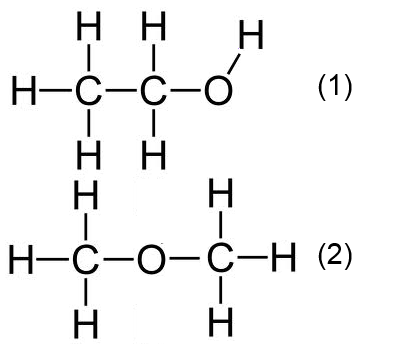# The structure of ethanol

## Determination of the molar mass

$2.3\; g$ of ethanol is vaporized and we note that its vapor occupies at $100^oC$ and $1\;atm$ a volume of $1.53\;L$. → Calculation

## Determination of the molecular formula

Elemental analysis revealed that ethanol contains $52,17\%\;C$ and $13,04\%\;H$ and oxygen. → Calculation

## Determination of the structural formula

The empirical formula allows only two possible structural formulas. → Which ?By reacting with an excess of sodium, $2.3\;g$ of ethanol provide $0.56\;L$ hydrogen under normal conditions. This allows to decide which of the above formulas is correct. → Explain !